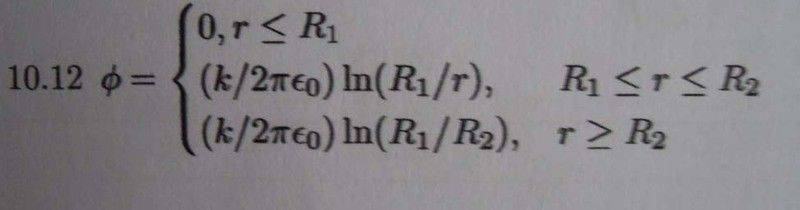# Electric field in a cylindrical capacitor

I like Serena
Homework Helper
It seems sum of enclosed charges is zero because k-k=0

Yes, it seems that way, and it is. ;)

Yes, it seems that way, and it is. ;)

However, when I think of it, it doesn't make sense.
I think there will be electric field outside the 2 capacitor.And from the answer of potential, the E electric isn't 0 in this case/.

#### Attachments

I like Serena
Homework Helper
Whaaaaat? You peeked! ;)

Ah well, did you try to calculate the gradient of phi outside the capacitor?
What is it?

Actually, I'm a bit surprised the book gives this as a solution.
You are free to select the integration constant in this problem, but usually it is taken such that the potential is zero at infinity.
Here they have chosen to have the potential zero inside the inner cylinder.

Btw, now that I see the solution, I suddenly realize the volume integral you calculated is wrong. Sorry.
You calculated the entire volume and assumed the charge density was constant everywhere and equal to k.
But it isn't. The charge is k per meter just on the outside of the cylinder.

To be clear:
For a cylinder of radius r and height delta z, with r between R1 and R2, the calculation is:
$$\iint\kern-1.4em\bigcirc\kern.7em E \cdot dA = \iiint \frac \rho {\epsilon_0} dV$$
$$E_r(r) \cdot 2 \pi r \Delta z = \frac k {\epsilon_0} \Delta z$$

Last edited:
Whaaaaat? You peeked! ;)

Ah well, did you try to calculate the gradient of phi outside the capacitor?
What is it?

Actually, I'm a bit surprised the book gives this as a solution.
You are free to select the integration constant in this problem, but usually it is taken such that the potential is zero at infinity.
Here they have chosen to have the potential zero inside the inner cylinder.

Btw, now that I see the solution, I suddenly realize the volume integral you calculated is wrong. Sorry.
You calculated the entire volume and assumed the charge density was constant everywhere and equal to k.
But it isn't. The charge is k per meter just on the outside of the cylinder.

To be clear:
For a cylinder of radius r and height delta z, with r between R1 and R2, the calculation is:
$$\iint\kern-1.4em\bigcirc\kern.7em E \cdot dA = \iiint \frac \rho \epsilon_0 dV$$
$$E_r(r) \cdot 2 \pi r \Delta z = \frac k \epsilon_0 \Delta z$$

Oh! I suddenly know why.
The electric field out the outer cylinder is zero.
However it doesn't imply that the potential is zero.
Instead, it means no change of potential.

Since at r=R2, the potential=$\frac{kln(R1/R2)}{2\pi \epsilon_0}$
Thus the potential outside the cylinder is still $\frac{kln(R1/R2)}{2\pi \epsilon_0}$

Am I right?

I like Serena
Homework Helper
Oh! I suddenly know why.
The electric field out the outer cylinder is zero.
However it doesn't imply that the potential is zero.
Instead, it means no change of potential.

Since at r=R2, the potential=$\frac{kln(R1/R2)}{2\pi \epsilon_0}$
Thus the potential outside the cylinder is still $\frac{kln(R1/R2)}{2\pi \epsilon_0}$

Am I right?

Yep.
The potential (outside the outer cylinder) does not depend on any coordinate, so its derivative (the electric field) is zero.

In fact I have answered that question, and we are now page 2.
Can you help me to check if I get the correct answer?

...Sorry I post it on page 1 but it is now on page 2...I don't know why...
It seems all questions are solved :-)

Thanks everyone!